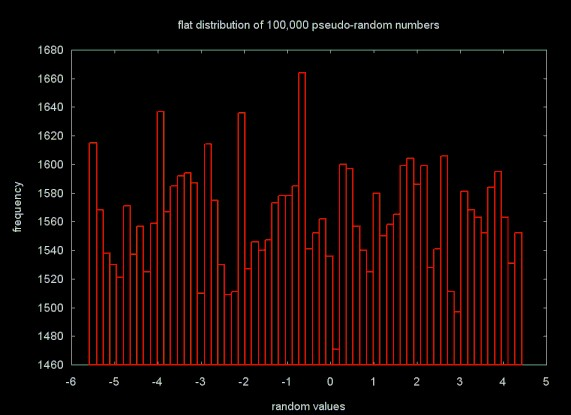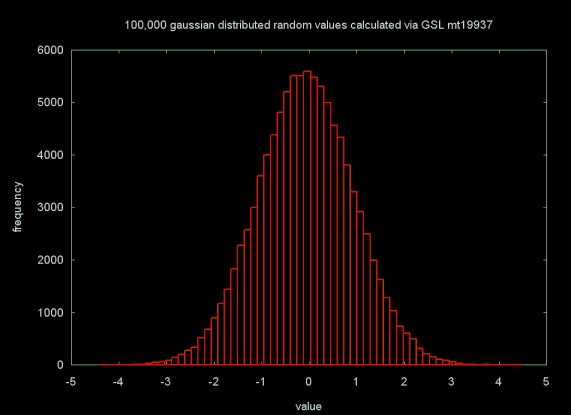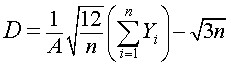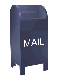## Pseudo-random Numbers

### Pseudo-random Numbers and Determinism

A true random value is generated by a physical process like a dice throw, counting the particles emitted by the decay of a radioactive element.

Pseudo-random numbers are generated by software functions. They are referred to as "pseudo-random" because the sequence of numbers is deterministic. Given a particular function and a "seed" value, the same sequence of numbers will be generated by the function. If the pseudo-random number generation function is well designed, the sequence of numbers will appear to be statistically random. However, there is no question that these numbers were generated by a deterministic process (e.g., the pseudo-random number function). The repeatability of the pseudo-random number sequence can be useful for debugging and simulation, since the program will calculate the same result every time it is run (which would not be true if a true random number generator were used). To avoid always writing "pseudo-random number", "random number" will be used below, with the understanding that I am referring to pseudo-random numbers.

### Flat, or uniformly distributed random numbers

The pseudo-random number generation functions available in most standard computer math libraries generate random numbers with a uniform distribution. If many "random" numbers are drawn from a particular region (say [0,1), where the value, v is in the range 0 <= v < 1) and a histogram plot is generated from the result, the histogram will form a block. This is shown below, where random numbers are generated in the range [5.5, 4.5):### Gaussian Random Numbers

A number of applications, including the generation of Brownian random walks, require random numbers that fall into a Gaussian distribution (e.g., a bell curve). An example of 100,000 random numbers in a uniform Gaussian distribution (where there is a mean of 0 and a standard deviation of 1) is shown below:### Converting a flat distribution to a Gaussian distribution

In Chaos and Fractals Peitgen et al propose a simple equation for converting random numbers with a uniform disribution into random numbers with a Gaussian distribution. They write:

In fact, the Gaussian distribution arises in all cases where independent and simpilar (i.e. identically distributed) random events are summed up or averaged. This is the context of an important mathematical theorem called the central limit theorem.

An example of a "random event" would be a throw of six, six sided dice. The value on the dice are added, yielding a value from 6 to 36. If 10,000 throws are made, the distribution of values will fall in a Gaussian curve. The principle of adding multiple random events is used by Peitgen et al to convert the values produced by a uniform random number generator into an approximation of a Gaussian distribution using the formula below:• D: a Gaussian random number

• A: the upper limit of the random number generator, which returns numbers 0, 1, ... A

• n: number of independent events (e.g., dice)

• Y1, Y2, ... Yn: results of an independent event (e.g., a dice throw)

A more rigorous method for generating Gaussian random numbers is published on Taygeta Scientific Inc.'s web page, written by Dr. Everett (Skip) F. Carter Jr., Generating Gaussian Random Numbers. Dr. Carter uses the polar form of the Box-Muller transform (OK, I've never heard of the Box-Muller transform either). The material below is copied from Dr. Carter's web page below:

```  // from http://www.taygeta.com/random/gaussian.html
// Algorithm by Dr. Everett (Skip) Carter, Jr.

float x1, x2, w, y1, y2;

do {
x1 = 2.0 * ranf() - 1.0;
x2 = 2.0 * ranf() - 1.0;
w = x1 * x1 + x2 * x2;
} while ( w >= 1.0 );

w = sqrt( (-2.0 * ln( w ) ) / w );
y1 = x1 * w;
y2 = x2 * w;
```

where ranf() is the routine to obtain a random number uniformly distributed in [0,1).

The function outlined above generates Gaussian random numbers in pairs (e.g., y1, y2). A function that returns a single random number would only calculate y1 or y2.

### High Quality Random Numbers

For many applications high quality random numbers, which are random in all bits and have a very large repeat period, are important. The answers obtained should not be a result of artifacts in the random number generator. Sadly, there does not seem to be a standard source for high quality random numbers in C/C++. The POSIX rand() function is notoriously poor. The random() function available on UNIX, freeBSD and Linux is a better choice, but I was unable to find a port of this code to Windows. The Boost library for C++ also provides random number generator support. But I did not find the Boost random number algorithms as clear or well documented the GNU Scientific Library (GSL).

The GSL provides a great solution to the problem of portable, high quality random number support. The GSL is portable on all major systems (UNIX, Linux and Windows) and provides random number suport that is better than the standard UNIX random() function. The Windows version is available as a precompiled Visual C++ library, packaged in a Windows self installer. While I was able to use this binary release, it is not clear to me that all symbols in the library are properly exported, since some of the code examples would not link properly (I could not resolve the builtin variables for the random number generator types).

The GSL provides a wide variety of random number functions and random number distributions. The library also includes support for a variety of other math functions, including basic statistics, linear algebra, the Fast Fourier Transform. As with all GNU software, the only limitation is that the software may not be included in any software you sell, unless you make the source code for your software available (see the copyleft license included along with the GSL).

I discovered the GSL after I read Everett Carter's web page (referenced above). As It turns out, the GSL uses a version of the Box-Muller transform as well in calculating a random Gaussian distribution. The function is included below. The comments are in the original.

```From the GNU Scientific Library, src/randist/gauss.c

/* Of the two methods provided below, I think the Polar method is more
* efficient, but only when you are actually producing two random
* deviates.  We don't produce two, because then we'd have to save one
* in a static variable for the next call, and that would screws up
* re-entrant or threaded code, so we only produce one.  This makes
* the Ratio method suddenly more appealing.  There are further tests
* one can make if the log() is slow.  See Knuth for details */

/* Both methods pass the statistical tests; but the polar method
* seems to be a touch faster on my home Pentium, EVEN though we
* are only using half of the available random deviates!
*/

/* Polar (Box-Mueller) method; See Knuth v2, 3rd ed, p122 */

double
gsl_ran_gaussian (const gsl_rng * r, const double sigma)
{
double x, y, r2;

do
{
/* choose x,y in uniform square (-1,-1) to (+1,+1) */

x = -1 + 2 * gsl_rng_uniform (r);
y = -1 + 2 * gsl_rng_uniform (r);

/* see if it is in the unit circle */
r2 = x * x + y * y;
}
while (r2 > 1.0 || r2 == 0);

/* Box-Muller transform */
return sigma * y * sqrt (-2.0 * log (r2) / r2);
}
```

If gsl_ran_gaussian is called with the sigma argument equal to 1.0, it will return a uniform Gaussian distribution (e.g., mean = 0, StdDev = 1).

### C++ Source Code

I have written a simple C++ base class which abstracts away the details of the GSL setup and cleanup. The C++ code which can be downloaded here includes two subclasses which support flat and gaussian distribution. I have included a simple test program and a class which supports histogram creation (which was used to generate the plots above). A Makefile, for Microsoft NMAKE is included to build the software. It should not be too difficult to convert this to a UNIX Makefile. The software is in tar format. You can download a copy of tar for Win32 here. An even better choice is to install the UNIX Cygwin shell and software tools for Windows, available from RedHat.

rand.tar

This archive will unpack into two directories: histo and rand. In order to compile this software you will need to install the GNU Scientific Library. The Windows Makefile is written for Visual C++ (I tested it on Visual C++ 6.0). Change the paths in the Makefile to correspond to the set-up on your local system. To build the random number generator test program go into the rand directory and type nmake.

Ian Kaplan
January 2003
Revised: